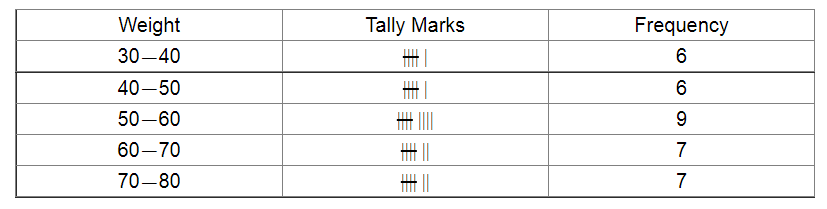# Construct a frequency table for the following weights (in gm) of 35 mangoes using the equal class intervals,

Question:

Construct a frequency table for the following weights (in gm) of 35 mangoes using the equal class intervals, one of them is 40-50 (45 not included):

30, 40, 45, 32, 43, 50, 55, 62, 70, 70, 61, 62, 53, 52, 50, 42, 35, 37, 53, 55, 65, 70, 73, 74, 45, 46, 58, 59, 60, 62, 74, 34, 35, 70, 68.

(i) What is the class mark of the class interval 40-45?

(ii) What is the range of the above weights?

(iii) How many classes are there?

Solution:

The frequency table for the given weights (in gm) of 35 mangoes is given below:(i) Class mark for the class interval $40-45$ :

Class mark $=\frac{40+45}{2}=\frac{85}{2}=42.5$

(ii) Range of the above weights:

Range $=$ Highest value $-$ Lowest value

$=74-30$

$=44$

(iii) There are 5 classes $(30-40,40-50,50-60,60-70,70-80)$.